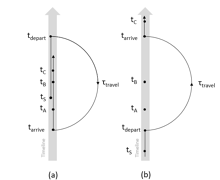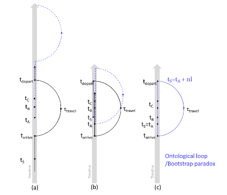67 Pages

Ripple dynamics is a topic studied in Temporal continuum dynamics. It is a model that describes the dynamics of time travel involving mutable histories in terms of the dynamics of ripple effects. (Some paragraph To be added once the bottleneck is solved)

Bottleneck=no known mechanism in GR and QFT to allow change in spacetime, not just spatial topology. Discontinuity issues with fields in spacetime introduced by mutable history time travel (Credits: Samuel Lereah)

## Ripple effect

A ripple effect is an undulation in a timeline, usually due to a history changing event, which results in the timeline to oscillate between multiple versions of histories (sometimes discontinuously). Usually a ripple takes the form of a pulse. However in some scenarios, a continuum of histories can be carried by the ripple and thus allowing the ripple to have a phase velocity like a wavepacket.

Ripples typically propagates toward the future direction of a timeline. Due to time travel events, however, it can end up emerging from the past.

## Newtonian ripple dynamics

### Inertial frames

#### One time travelFigure 1: Schematics of two fundamental units of time travel under the Ripple Dynamics Model for 3 ripples produced by the same source S and felt by the events A, B, C respectively. Variables explained in main article. (a) Past time travel, (b) Future time travel.

Consider 3 ripples with the same sourceand events where they hit. Then the (average) group velocities of the ripples, as seen from the time traveler's frame are obtained byThat is, the group velocity is the average time transverses by the ripple, per ticks of the clocks in the time traveler's frame (Thus unlike ordinary group velocities, it is unitless). Therefore for, the group velocity of the ith ripple is given bywhereandare the time of departure from the timeline due to a time travelling event, the time of arrival due to the same time travelling event and the (proper) time elapsed due to the time travel respectively. Let, then the ripple group velocity formula takes the more compact formNow ifthenand hence in (1). This is only true if(taking account the fact that the path of the ripple in the timeline may revisit a point moreFigure 2: Schematics illustrating the special case ti + I = tS. (a) As shown, it is impossible for tA to connect to tS when I is added to tA. (b) There is also in general no solution for ti + I = tS in backward time travel either. (c) An ontological loop represents a solution to ti + I = tS as tA and tS joined up perfectly after adding I.

than once due to the looping back introduced by the topology of backward time travel). In addition, (2) only holds if the path revisit itself at least once which means for future time travel it is impossible to have (2) satisfied. For the case involving just one backward time travel, the special case of (2) whereandcoincides corresponds to an ontological loop, which has the property that there is no well defined source event. Because of that, it is meaningless to talk about the ripples' velocity in such case. As seen from Figure 2, (2) can be generalised for this special case for any integersasForthe velocities are given byTherefore the velocities will depend on the delayandbetween the source and the event where their effects are perceived in the frame (with the limiting case of infinity if the source occurs arbitrarily close to the event, corresponding to an instantaneous history change felt by all points of the timeline).

If we instead rearranging (1) and assuming the time travelers are in the same inertial frame as the eventsand, thenwithand we getNote ifthen, which represents a future time travel that took the same amount of time as the time interval of the departure and arrival points in the timeline (Recall thatsince the (proper) time elapsed always increases, except for the highly unusual scenario involving Star Trek's antitime, where it is some frame where the proper time decrements rather than increments. Thereforemeans, which is the same as not time travelling at all). Thereforeand henceUsing this in (1) forwe obtain the other velocities asThis means the jth ripple depends on the ith ripple's velocity and the time displacementfrom the ith event to the jth event if the ripples share the same source.

##### Limiting cases

If the time travel involve events that are e.g. years apart, but the events where the ripple hits and the sources are only a few minutes apart, thenwill dominate other terms. HenceOr in terms of the time where the source occursIf the time separation between the jth and ith event decreases, the corresponding ripples will have similar velocity. Therefore, the relative magnitude of the ripples' velocities will give a measure on the time separation between the two events caused by the same source.

It is easy to see that in general for such cases, the ripple will be superluminal. The approach to the implications on such phenomenon is disputed. One approach suggests this highlighted the limitation of describing ripples under the simplification of Newtonian mechanics and relativistic treatment of the dynamics is required to resolve this issue. Another suggests that there is no issue with superluminality given how backward time travel phenomenon is already a causality violating phenomenon at least in the local timeline and that there are no indications that suggest ripples, which are oscillation of different versions of spacetime, should behave like other matter and energy.

### Accelerated frames

(To be calculated)

## Classical mechanics formulation

(To be calculated)

## Relativistic ripple dynamics

(Requires solving the Bottleneck)

## Ripple dynamics in warped spacetime

(Requires solving the Bottleneck)

## Quantum ripple field theory

(Requires solving the Bottleneck (and possibly not even a well define notion)

Community content is available under CC-BY-SA unless otherwise noted.